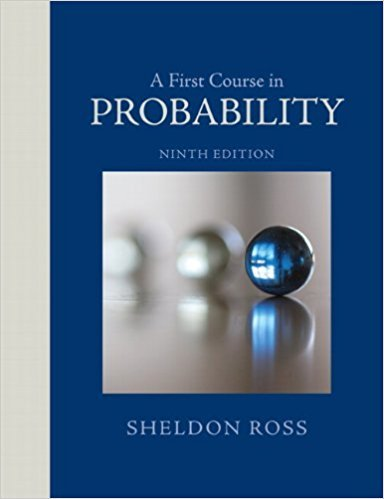×
Get Full Access to A First Course In Probability - 9 Edition - Chapter 3 - Problem 82p
Get Full Access to A First Course In Probability - 9 Edition - Chapter 3 - Problem 82p

×

# A and B flip coins. A starts and continues flipping untilISBN: 9780321794772 63

## Solution for problem 82P Chapter 3

A First Course in Probability | 9th Edition

• Textbook Solutions
• 2901 Step-by-step solutions solved by professors and subject experts
• Get 24/7 help from StudySoup virtual teaching assistantsA First Course in Probability | 9th Edition

4 5 1 316 Reviews
11
2
Problem 82P

Problem 82P

A and B flip coins. A starts and continues flipping until a tail occurs, at which point B starts flipping and continues until there is a tail. Then A takes over, and so on. Let P1 be the probability of the coin landing on heads when A flips and P2 when B flips. The winner of the game is the first one to get

(a) 2 heads in a row;

(b) a total of 2 heads;

(c) 3 heads in a row;

(d) a total of 3 heads.

In each case, find the probability that A wins.

Step-by-Step Solution:

Solution

Step 1 of 4

Given that A and B flip the coins

A start flipping the coin until tail occur then B takes over

B start flipping the coin until tail occur then A takes over

When A flips the coinis the probability of getting head

When B flips the coinis the probability of getting head

a) We have to find the probability of A wins when 2 heads in a row

Here we want the probability that A gets 2 heads before B gets

To find this

let A be the event that A gets 2 heads in row before B where A goes first means A wins

let B be the event that B gets 2 heads in row before A where B goes first means B wins

To find P(A) by conditioning on the output of the first 2 flips

To find that let us introduce the events that what is chance of happening in the first 2 flips

Letare the events of A’s coin lands heads up or tails up

And in the same way

Letare the events of B’s coin lands heads up or tails up

Now the conditioning on the first 2 flips===…………(1)

Herebecause if a flipsthe coin goes to B

In the above expression we needNow the conditioning on the first 2 flips===Substitute this expression in the equation (1)  and get the value ofNow=Step 2 of 4

Step 3 of 4

##### ISBN: 9780321794772

Unlock Textbook Solution